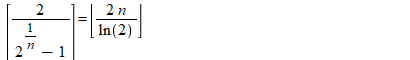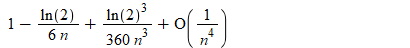:

## An elementary problem

Maple

Here is a very nice (but not easy) elementary problem.
The equality
ceil(2/(2^(1/n)-1)) = floor(2*n/ln(2));is not an identity, it does not hold for each positive integer n.
How to find such a number?

[This is a re-post, because the original vanished when trying a conversion Question-->Post]

The problem appears in the recent book:
Richard P. Stanley - Conversational Problem Solving. AMS, 2020.

The problem is related to a n-dimensional tic-tac-toe game. The first counterexample (2000) was wrong due to a multiprecision arithmetic error.
The  author of the book writes
"To my knowledge, only eight values of n are known for which the equation fails,
and it is not known whether there are infinitely many such values",

but using Maple it will be easy to find more.

A brute-force solution is problematic because the smallest counterexample is > 7*10^14.

```restart;
a := 2/(2^(1/n)-1): b := 2*n/ln(2):
asympt(b-a, n);
```It results:  b - a → 1 (for n →oo);
So, to have a counterexample, b must be close to an integer
m ≈ 2*n/ln(2)  ==> n/m ≈ ln(2)/2

The candidates for n/m will be obviously the convergents of the continued fraction of the irrational number ln(2)/2.

```convert(ln(2)/2, confrac, 200, 'L'):
Digits:=500:
for n in numer~(L[3..]) do
if not evalf(ceil(a)=floor(b)) then printf("n=%d\n", n) fi;
od:
```

n=777451915729368
n=140894092055857794
n=1526223088619171207
n=54545811706258836911039145
n=624965662836733496131286135873807507
n=1667672249427111806462471627630318921648499
n=36465374036664559522628534720215805439659141
n=2424113537313479652351566323080535902276508627
n=123447463532804139472316739803506251988903644272
n=97841697218028095572510076719589816668243339678931971
n=5630139432241886550932967438485653485900841911029964871
n=678285039039320287244063811222441860326049085269592368999
n=312248823968901304612135523777926467950572570270886324722782642817828920779530446911
n=5126378297284476009502432193466392279080801593096986305822277185206388903158084832387
n=1868266384496708840693682923003493054768730136715216748598418855972395912786276854715767
n=726011811269636138610858097839553470902342131901683076550627061487326331082639308139922553824778693815

So, we have obtained 16 counterexamples. The question whether there are an infinity of such n's remains open.﻿